#### You may also like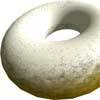### Doughnut

How can you cut a doughnut into 8 equal pieces with only three cuts of a knife?### Rectangle Tangle

The large rectangle is divided into a series of smaller quadrilaterals and triangles. Can you untangle what fractional part is represented by each of the shapes?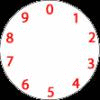### Dividing a Cake

Annie cut this numbered cake into 3 pieces with 3 cuts so that the numbers on each piece added to the same total. Where were the cuts and what fraction of the whole cake was each piece?

##### Age 7 to 11 Challenge Level: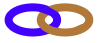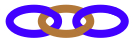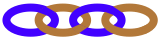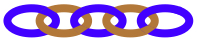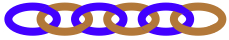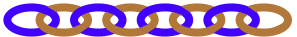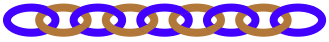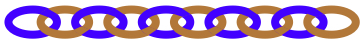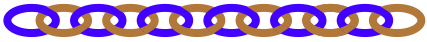Here are lots of small chains, each with a certain number of links.  The chains that we will use have these numbers of links:  2, 3, 4, 5, 6, 8, 9, 10 and 12. There are many of each size available.

Suppose we want to make a twenty-link chain from the sizes above, then we could use two of the ten-link small chains. Each ten would just be $\frac{1}{2}$ of the 20.
In this case we could write:

Half plus half makes one whole, or, $\frac{1}{2} + \frac{1}{2} = 1$
where the twenty-link chain is the ONE (1)

What other selection of chains could you put together to make the twenty-link chain, using only the sizes that you see at the top?
Try writing the fractions down in different ways.

Find as many different ways of making a twenty-link chain as you can.

What happens if you try to make a twenty-four-link chain?

Here is an example using a twelve-, an eight- and a four-link chain:

We could write:
$\frac{1}{2} + \frac{1}{3} + \frac{1}{6} = 1$
where the twenty-four-link chain is the ONE (1)

Or, we could write:
a half plus a third plus a sixth makes a one

How many ways can you find to make a twenty-four-link chain?

A very different one to try is a twenty-seven-link chain, so 27 is your ONE.  Have a go!

### Why do this problem?

This activity is an unusual context in which pupils can consolidate recognising, finding, naming and writing fractions.  The rich environment also gives them the opportunity to identify, name and write equivalent fractions of a given fraction, represented visually as a chain.  Furthermore, learners will be adding and subtracting fractions with the same denominators and denominators which are multiples of the same number.

### Possible approach

Having some practical resource available for children to use if they wish is key to making it accessible. You may be able to borrow links that can be joined to make a chain (perhaps from your Early Years setting), or you could make decorations from loops of paper.  Alternatively, you could use a rod of each number of connecting cubes that are the same colour for each length.

Have many examples of each size of chain and give the group the challenge of making a twenty-link chain. Depending on their experience of naming fractions, you could just use tens and fives  to begin with to help the pupils have a feel for them being halves and quarters of the twenty.

Once they are comfortable about referring to the small chains as fractions of the twenty-link chain then you can invite them to explore the many ways of making the twenty-link chain as a whole.

The first part of the activity focusing on the twenty-link chain could be a 'simmering task', so that learners consider it out of lesson time and contribute their ideas to a working Maths wall, for example.  You can then revisit that first part, perhaps even several times, before moving on to the twenty-four-link chain and the twenty-seven-link chain.

### Key questions

Tell me about the fractions you've decided to use.
Could you replace your half (or other suitable fraction) with two or three other small chains?

### Possible extension

Encourage learners to take three or four random small chains and put them together.  What fraction of the twenty/twenty-four/twenty-seven do they make?

For example, if you chose the 4, 6, 8 as $\frac{1}{5} + \frac{3}{10} + \frac{4}{10}$ you would have $\frac{9}{10}$ when twenty links is the whole 1.
The same three would be $\frac{1}{6} + \frac{1}{4} + \frac{1}{3}$ and you would have $\frac{3}{4}$ when twenty-four links is the whole 1.

### Possible support

Children who have difficulties with fine motor skills may need help manipulating the small chains.